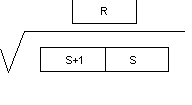## How to Find Square Root in PLC Programming

Dealing with the Process Parameters and Processing them is an integral part while making PLC Program for High End Process Application. Sometimes it happens that we need to find out the Square Root of a Particular Data. Omron PLC has got a very useful instruction for this purpose and we can directly calculate the Square root of a Value.Subscribe to Automation-Talk by Email.

Calculate Square Root of A Value in Omron PLC :-

Square Root of a particular Data Word can be directly calculated in Omron PLC with a simple instruction. The name of instruction is ROOT.
Syntax :- ROOT S R
Where :- S is the Source Data Word whose Square Root is to be calculated and R is the Result Data word where the result will be stored.
This Instruction computes the square root of the eight-digit contents of Sq and Sq+1 and places the result in R. The fractional portion is truncated.

The value of square root will be calculated according to the data present in following Data Word as shown in Below Picture.This instruction is very useful in handling and processing of Process Data.
Square Root Calculation PLC Program.